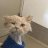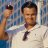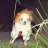# Balance of Power Trend Indicator for ThinkorSwim#### BenTen

Staff
VIP
This indicator will help you determine the strength of bulls and bears. It's similar to the default Balance Of Market Power in ThinkorSwim but with additional bells and whistles.
• Above the Zero line is bullish
• Below the Zero line is considered bearishCode:
``````# Balance of Power Trend
# Assembled by BenTen at useThinkScript.com

declare lower;

input EMA = 34;
input TEMA = 34;
input high_l = 0.1;
input low_l = -0.1;

def THL = if(high != low, high - low, 0.01);
def BullOpen = (high - open) / THL;
def BearOpen = (open - low) / THL;
def BullClose = (close - low) / THL;
def BearClose = (high - close) / THL;
def BullOC = if(close > open, (close - open) / THL, 0);
def BearOC = if(open > close, (open - close) / THL, 0);
def BullReward = (BullOpen + BullClose + BullOC) / 3;
def BearReward = (BearOpen + BearClose + BearOC) / 3;
def BOP = BullReward - BearReward;

def SmoothBOP = expAverage(BOP, EMA);
def xPrice = SmoothBOP;
def xEMA1 = expAverage(SmoothBOP, TEMA);
def xEMA2 = expAverage(xEMA1, TEMA);
def xEMA3 = expAverage(xEMA2, TEMA);
def nRes = 3 * xEMA1 - 3 * xEMA2 + xEMA3;
def SmootherBOP = nRes;

plot h = high_l;
plot l = low_l;
plot ZeroLine = 0;

plot s1 = SmoothBOP;
plot s2 = SmootherBOP;
plot s3 = SmootherBOP;

ZeroLine.SetDefaultColor(GetColor(3));
h.SetDefaultColor(GetColor(3));
l.SetDefaultColor(GetColor(3));``````

••poparhon, veerasareddy, diazlaz and 5 others
J

#### john3

##### Active member
2019 Donor
@BenTen Looks interesting, thank you. Is there a way to plot this as an upper study?

J

#### john3

##### Active member
2019 Donor
@BenTen If it isn't possible, I think it would be very useful to have an option to plot the crossing signals on an upper chart.

Thank you.#### BenTen

Staff
VIP
@john3 which crossover?

J

#### john3

##### Active member
2019 Donor
@john3 which crossover?

When the green/red lines cross and when the yellow line crosses the "channel" - when it crosses up/down both green/red lines.#### markos

##### Well-known member
VIPVery nice divergence clue that the gap up was low probability! Thanks @BenTen

•BenTen
J

#### john3

##### Active member
2019 Donor
@BenTen Just to clarify, when I said to make it plot as an upper study, I didn't mean the three lines (zero, -0.1, +0.1). I mean the "channel," the green/red lines and the yellow line, so it would be the S1, S2, and S3 plots.#### markos

##### Well-known member
VIP
@BenTen Just to clarify, when I said to make it plot as an upper study, I didn't mean the three lines (zero, -0.1, +0.1). I mean the "channel," the green/red lines and the yellow line, so it would be the S1, S2, and S3 plots.
@john3 that would be changing the intent if the indicator. It is not meant as a s/r indicator.
TDA offers free tours if you haven't had yours yet. Have 10 questions ready to ask so that you can be shown what you want to see.

Last edited:
•BenTen
R

##### MemberVery nice divergence clue that the gap up was low probability! Thanks @BenTen
@markos could you please explain how you use/read this indicator? Like how the crossing over green and red line and yellow over the channel? thanks,

Last edited:
R

##### Member
@BenTen I understand the indicator above zero bullish, below zero bearish. How to I interpret the green, red and yellow lines? I think it is telling more as they trend downwards or upwards,#### BenTen

Staff
VIP
@RPrado Like @markos suggested, it can be used to find divergences. The red and yellow lines are 34 moving averages.

•D

#### diazlaz

##### Well-known member
2019 Donor
VIP
This indicator responses well in intraday timeframes.

•##### Member
Hi @BenTen , Found this version of the code which has a P/L. What can I do to limit the contract size to 1 and have it only calculate P/L results during the cash market open? Thanks!

Code:
``````# Balance of Power Trend
# Assembled by BenTen at useThinkScript.com

declare lower;

input EMA = 34;
input TEMA = 34;
input high_l = 0.1;
input low_l = -0.1;

def THL = if(high != low, high - low, 0.01);
def BullOpen = (high - open) / THL;
def BearOpen = (open - low) / THL;
def BullClose = (close - low) / THL;
def BearClose = (high - close) / THL;
def BullOC = if(close > open, (close - open) / THL, 0);
def BearOC = if(open > close, (open - close) / THL, 0);
def BullReward = (BullOpen + BullClose + BullOC) / 3;
def BearReward = (BearOpen + BearClose + BearOC) / 3;
def BOP = BullReward - BearReward;

def SmoothBOP = expAverage(BOP, EMA);
def xPrice = SmoothBOP;
def xEMA1 = expAverage(SmoothBOP, TEMA);
def xEMA2 = expAverage(xEMA1, TEMA);
def xEMA3 = expAverage(xEMA2, TEMA);
def nRes = 3 * xEMA1 - 3 * xEMA2 + xEMA3;
def SmootherBOP = nRes;

plot h = high_l;
plot l = low_l;
plot ZeroLine = 0;

plot s1 = SmoothBOP;
plot s2 = SmootherBOP;
plot s3 = SmootherBOP;
s1.SetDefaultColor(Color.DARK_GRAY);
#s2.SetDefaultColor(Color.BLUE);
s3.SetDefaultColor(Color.YELLOW);
#s2.SetStyle(Curve.SHORT_DASH);
s3.SetStyle(Curve.FIRM);
#s2.SetLineWeight(3);
s3.SetLineWeight(3);

s2.SetDefaultColor(GetColor(5));
s2.SetPaintingStrategy(PaintingStrategy.HISTOGRAM);
s2.SetLineWeight(5);
s2.DefineColor("Positive and Up", Color.GREEN);
s2.DefineColor("Positive and Down", Color.DARK_GREEN);
s2.DefineColor("Negative and Down", Color.RED);
s2.DefineColor("Negative and Up", Color.DARK_RED);
s2.AssignValueColor(if s2 >= s3 then if s2 > s2 then s2.color("Positive and Up") else s2.color("Positive and Down") else if s2 < s2 then s2.color("Negative and Down") else s2.color("Negative and Up"));

ZeroLine.SetDefaultColor(GetColor(3));
h.SetDefaultColor(GetColor(3));
l.SetDefaultColor(GetColor(3));

#Vertical Lines
def short = s2 < s3 and s2 > s3;
def long = s2 > s3 and s2 < s3;

################################################################################################
input PandL_Label = Yes;

def orderDir = CompoundValue(1, if  s2 < s3 then 1 else if s2 > s3 then -1 else orderDir, 0);
def isOrder = orderDir crosses 0;

def orderCount = CompoundValue(1, if IsNaN(isOrder) then 0 else if isOrder then orderCount + 1 else orderCount, 0);

def noBar = IsNaN(open[-1]);

def orderPrice = if isOrder then if noBar then close else open[-1] else orderPrice;
def profitLoss = if !isOrder or orderCount == 1
then 0
else if orderDir < 0 then orderPrice - orderPrice
else if orderDir > 0 then orderPrice - orderPrice else 0;
#else if orderDir > 0 then orderPrice - orderPrice
#else if orderDir < 0 then orderPrice - orderPrice else 0;
#def profitLoss = if !isOrder or orderCount == 1 then 0 else orderPrice - orderPrice;
def profitLossSum = CompoundValue(1, if IsNaN(isOrder) then 0 else if isOrder then profitLossSum + profitLoss else profitLossSum, 0);

def orderWinners = CompoundValue(1, if IsNaN(isOrder) then orderWinners else if orderCount > 1 and profitLoss > 0 then orderWinners + 1 else orderWinners, 0);

AddLabel(PandL_Label, orderCount + " orders (" + AsPercent(orderWinners / orderCount) + ") | P/L " + AsDollars((profitLossSum / TickSize()) * TickValue()), if profitLossSum > 0 then Color.GREEN else if profitLossSum < 0 then Color.RED else Color.GRAY);``````

T

#### Tito

##### New member
Hi everyone. I am relatively new at trading and at TOS and in checking indicators, I have been needing an indicator that does not exist.. Balance of Market Power is the closest I found but I don't think the formula is right ... I came up with my own formula and I am trying to build a script modifying a little the preexisting script for this indicator that exist already in TOS. I don't know if anyone can orient me a little.
Thanks very much#### BenTen

Staff
VIP
@Tito It would be best to include the script as well.

T

#### Tito

##### New member
@Tito It would be best to include the script as well.
Thanks for answering Ben.. I don't have the script. I don't know where or how to get it. I just saw this script on this trend but is a little more complex than what I am looking for.. What I think is that the formula Balance of Power = (Close price – Open price) / (High price – Low price) is not very well thought out , although the general idea is good. I want to replace it by ((closing price -low price)- (high price-closing price))/ (High price -Low price)

T

#### Tito

##### New member
The idea would be to add the upper wick to the bearish element and to add the lower wick to the bullish so that the calculation would be more precise... Especially I would like to know this in areas of consolidation..

The regular formula...
Let me give an example
If we have a candle that has CP=4, OP=2, High=5, Low=2 then BAP=(4-2)/(5-2)=2/3
but...
If we have a candle that has CP=4, OP=2, High=4, Low=1 then BAP=(4-2)/(4-1)=2/3
So... Even though the wick is located in different extreme of the candle, the result remains the same which is somewhat misleading ..

L

#### lindosskier

##### Member
Hi @BenTen , is there any way to convert the "Balance of Market Power 14" from Trading View, into a TOS indicator? Thank you.On Balance Volume Scanner Questions 12TD Ameritrade now requires \$500 minimum balance for real time quotes paper trading Playground 9chart of account balance history? Questions 1